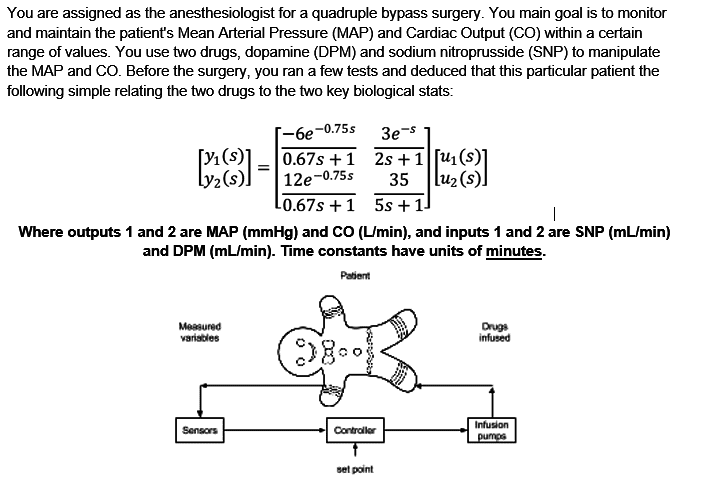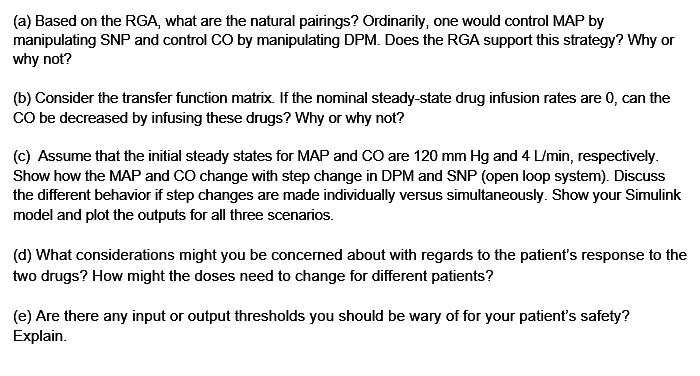# Multiple input-output control

• gfd43tg

Gold Member

## Homework Statement## The Attempt at a Solution

(a) I find the steady state values (s=0)
Code:
syms s
k11 = -6;
k12 = 3;
k21 = 12;
k22 = 35;

G = [k11 k12; k21 k22];
Ghat = [k22/(k11*k22-k12*k21) -k12/(k11*k22-k12*k21); -k21/(k11*k22-k12*k21) k11/(k11*k22-k12*k21)];

Lam = G*Ghat
Lam

Lam =

1  0
0  1
So I know that input 1 goes with output 1, and input 2 goes with output 2.

(b) I am not sure how I should do this, but I look at steady state values
Code:
G =

-6  3
12  35
But I don't really know how steady state infusion rates can be zero, yet you can increase Cardiac output by infusion? I don't understand how to solve this question.